### Mathematical Description of NPSC

A network consists of a collection of nodes joined by a collection of arcs. The arcs connect nodes and convey flow of one or more commodities that are supplied at supply nodes and demanded at demand nodes in the network. Each arc has a cost per unit of flow, a flow capacity, and a lower flow bound associated with it. An important concept in network modeling is conservation of flow. Conservation of flow means that the total flow in arcs directed toward a node, plus the supply at the node, minus the demand at the node, equals the total flow in arcs directed away from the node.

Often all the details of a problem cannot be specified in a network model alone. In many of these cases, these details can be represented by the addition of side constraints to the model. Side constraints are linear functions of arc variables (variables containing flow through an arc) and nonarc variables (variables that are not part of the network). The data for a side constraint consist of coefficients of arcs and coefficients of nonarc variables, a constraint type (that is,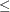,, or
) and a right-hand-side value (rhs). A nonarc variable has a name, an objective function coefficient analogous to an arc cost, an upper bound analogous to an arc capacity, and a lower bound analogous to an arc lower flow bound.

If a network component of NPSC is removed by merging arcs and nonarc variables into a single set of variables, and if the flow conservation constraints and side constraints are merged into a single set of constraints, the result is an LP problem. PROC INTPOINT will automatically transform an NPSC problem into an equivalent LP problem, perform the optimization, then transform the problem back into its original form. By doing this, PROC INTPOINT finds the flow through the network and the values of any nonarc variables that minimize the total cost of the solution. Flow conservation is met, flow through each arc is on or between the arc’s lower flow bound and capacity, the value of each nonarc variable is on or between the nonarc’s lower and upper bounds, and the side constraints are satisfied.

Note that, since many LPs have large embedded networks, PROC INTPOINT is an attractive alternative to the LP procedure in many cases. Rather than formulating all problems as LPs, network models remain conceptually easy since they are based on network diagrams that represent the problem pictorially. PROC INTPOINT accepts the network specification in a format that is particularly suited to networks. This not only simplifies problem description but also aids in the interpretation of the solution. The conversion to and from the equivalent LP is done behind the scenes by the procedure.

If a network programming problem with side constraints hasnodes,arcs,nonarc variables, andside constraints, then the formal statement of the problem solved by PROC INTPOINT is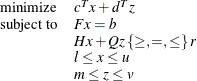where

•is the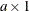arc variable objective function coefficient vector (the cost vector)

•is thearc variable value vector (the flow vector)

•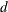is the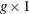nonarc variable objective function coefficient vector

•is thenonarc variable value vector

•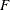is thenode-arc incidence matrix of the network, where

•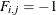if arcis directed from node•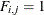if arcis directed toward node•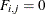otherwise

•is the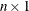node supply/demand vector, where

•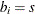if nodehas supply capability ofunits of flow

•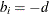if nodehas demand ofunits of flow

•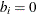if nodeis a transshipment node

•
is the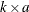side constraint coefficient matrix for arc variables, where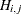is the coefficient of arcin theth side constraint

•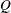is the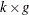side constraint coefficient matrix for nonarc variables, where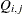is the coefficient of nonarcin theth side constraint

•is the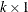side constraint right-hand-side vector

•is thearc lower flow bound vector

•is thearc capacity vector

•is thenonarc variable lower bound vector

•is thenonarc variable upper bound vector

The INTPOINT procedure can also be used to solve an unconstrained network problem, that is, one in which,,,, anddo not exist. It can also be used to solve a network problem with side constraints but no nonarc variables, in which case,, anddo not exist.